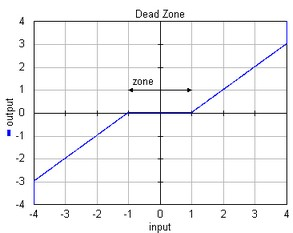﻿ 20-sim webhelp > Library > Signal > Block Diagram Non-Linear > deadzone

## Library

Signal\Block Diagram Non-Linear

## Use

Domains: Discrete, Continuous. Size: 1-D. Allowed in: Block Diagrams.

## Description

This model generates zero output when the input is within a specified region, called the dead zone. Otherwise the output equals the input plus or minus half the zone.

output = input + zone/2; (input < -zone/2)

output = 0; (-zone/2 <= input <= zone/2)

output = input - zone/2; (input > zone/2)Inputs

Description

input

output

zone